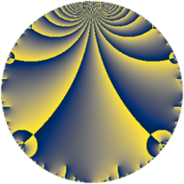# Properties

 Label 2023.4.aLevel $2023$ Weight $4$ Character orbit 2023.a Rep. character $\chi_{2023}(1,\cdot)$ Character field $\Q$ Dimension $407$ Newform subspaces $22$ Sturm bound $816$ Trace bound $6$

# Related objects

## Defining parameters

 Level: $$N$$ $$=$$ $$2023 = 7 \cdot 17^{2}$$ Weight: $$k$$ $$=$$ $$4$$ Character orbit: $$[\chi]$$ $$=$$ 2023.a (trivial) Character field: $$\Q$$ Newform subspaces: $$22$$ Sturm bound: $$816$$ Trace bound: $$6$$ Distinguishing $$T_p$$: $$2$$, $$3$$

## Dimensions

The following table gives the dimensions of various subspaces of $$M_{4}(\Gamma_0(2023))$$.

Total New Old
Modular forms 630 407 223
Cusp forms 594 407 187
Eisenstein series 36 0 36

The following table gives the dimensions of the cuspidal new subspaces with specified eigenvalues for the Atkin-Lehner operators and the Fricke involution.

$$7$$$$17$$FrickeDim.
$$+$$$$+$$$$+$$$$104$$
$$+$$$$-$$$$-$$$$100$$
$$-$$$$+$$$$-$$$$95$$
$$-$$$$-$$$$+$$$$108$$
Plus space$$+$$$$212$$
Minus space$$-$$$$195$$

## Trace form

 $$407q - q^{2} - 6q^{3} + 1649q^{4} - 62q^{6} - 7q^{7} + 15q^{8} + 3759q^{9} + O(q^{10})$$ $$407q - q^{2} - 6q^{3} + 1649q^{4} - 62q^{6} - 7q^{7} + 15q^{8} + 3759q^{9} - 128q^{10} - 60q^{11} - 34q^{12} - 28q^{13} + 7q^{14} + 96q^{15} + 6561q^{16} - 233q^{18} + 110q^{19} - 320q^{20} - 14q^{21} + 224q^{22} - 64q^{23} - 1158q^{24} + 9717q^{25} + 44q^{26} - 300q^{27} + 49q^{28} - 694q^{29} + 804q^{30} - 44q^{31} + 919q^{32} - 168q^{33} + 168q^{35} + 17437q^{36} + 234q^{37} + 982q^{38} + 640q^{39} + 724q^{40} - 542q^{41} + 14q^{42} - 572q^{43} + 56q^{44} + 112q^{45} - 168q^{46} + 1284q^{47} + 3162q^{48} + 19943q^{49} + 1409q^{50} + 232q^{52} - 422q^{53} + 1728q^{54} + 352q^{55} - 105q^{56} - 1180q^{57} - 1630q^{58} + 582q^{59} + 2884q^{60} - 568q^{61} + 4076q^{62} + 273q^{63} + 25633q^{64} - 384q^{65} + 3392q^{66} - 816q^{67} - 1000q^{69} - 616q^{70} - 232q^{71} + 455q^{72} + 838q^{73} - 42q^{74} - 922q^{75} - 2934q^{76} + 896q^{77} + 940q^{78} - 3160q^{79} - 720q^{80} + 33627q^{81} + 2842q^{82} - 618q^{83} + 98q^{84} + 2176q^{86} - 36q^{87} - 2860q^{88} - 4274q^{89} - 700q^{90} - 532q^{91} + 5512q^{92} + 2840q^{93} - 1888q^{94} - 1176q^{95} - 1070q^{96} + 194q^{97} - 49q^{98} - 6396q^{99} + O(q^{100})$$

## Decomposition of $$S_{4}^{\mathrm{new}}(\Gamma_0(2023))$$ into newform subspaces

Label Dim. $$A$$ Field CM Traces A-L signs $q$-expansion
$$a_2$$ $$a_3$$ $$a_5$$ $$a_7$$ 7 17
2023.4.a.a $$1$$ $$119.361$$ $$\Q$$ None $$-1$$ $$2$$ $$-16$$ $$7$$ $$-$$ $$+$$ $$q-q^{2}+2q^{3}-7q^{4}-2^{4}q^{5}-2q^{6}+\cdots$$
2023.4.a.b $$1$$ $$119.361$$ $$\Q$$ None $$-1$$ $$6$$ $$20$$ $$7$$ $$-$$ $$+$$ $$q-q^{2}+6q^{3}-7q^{4}+20q^{5}-6q^{6}+\cdots$$
2023.4.a.c $$1$$ $$119.361$$ $$\Q$$ None $$3$$ $$-1$$ $$6$$ $$-7$$ $$+$$ $$+$$ $$q+3q^{2}-q^{3}+q^{4}+6q^{5}-3q^{6}-7q^{7}+\cdots$$
2023.4.a.d $$1$$ $$119.361$$ $$\Q$$ None $$3$$ $$1$$ $$-6$$ $$7$$ $$-$$ $$-$$ $$q+3q^{2}+q^{3}+q^{4}-6q^{5}+3q^{6}+7q^{7}+\cdots$$
2023.4.a.e $$3$$ $$119.361$$ 3.3.2429.1 None $$-1$$ $$-5$$ $$19$$ $$21$$ $$-$$ $$+$$ $$q-\beta _{1}q^{2}+(-1-\beta _{1}+\beta _{2})q^{3}+(2+\beta _{1}+\cdots)q^{4}+\cdots$$
2023.4.a.f $$4$$ $$119.361$$ 4.4.68557.1 None $$-2$$ $$7$$ $$9$$ $$-28$$ $$+$$ $$+$$ $$q+(-1-\beta _{2})q^{2}+(2+\beta _{1}+\beta _{3})q^{3}+\cdots$$
2023.4.a.g $$7$$ $$119.361$$ $$\mathbb{Q}[x]/(x^{7} - \cdots)$$ None $$4$$ $$-5$$ $$-35$$ $$49$$ $$-$$ $$+$$ $$q+(1-\beta _{1})q^{2}+(-1+\beta _{2}+\beta _{3})q^{3}+\cdots$$
2023.4.a.h $$9$$ $$119.361$$ $$\mathbb{Q}[x]/(x^{9} - \cdots)$$ None $$2$$ $$-11$$ $$3$$ $$-63$$ $$+$$ $$+$$ $$q+\beta _{1}q^{2}+(-1-\beta _{5})q^{3}+(4+\beta _{2})q^{4}+\cdots$$
2023.4.a.i $$11$$ $$119.361$$ $$\mathbb{Q}[x]/(x^{11} - \cdots)$$ None $$-7$$ $$-2$$ $$2$$ $$-77$$ $$+$$ $$-$$ $$q+(-1+\beta _{1})q^{2}-\beta _{3}q^{3}+(4-\beta _{1}+\beta _{2}+\cdots)q^{4}+\cdots$$
2023.4.a.j $$11$$ $$119.361$$ $$\mathbb{Q}[x]/(x^{11} - \cdots)$$ None $$-7$$ $$2$$ $$-2$$ $$77$$ $$-$$ $$+$$ $$q+(-1+\beta _{1})q^{2}+\beta _{3}q^{3}+(4-\beta _{1}+\beta _{2}+\cdots)q^{4}+\cdots$$
2023.4.a.k $$12$$ $$119.361$$ $$\mathbb{Q}[x]/(x^{12} - \cdots)$$ None $$3$$ $$-3$$ $$12$$ $$84$$ $$-$$ $$-$$ $$q+\beta _{1}q^{2}+\beta _{4}q^{3}+(5+\beta _{1}+\beta _{2})q^{4}+\cdots$$
2023.4.a.l $$12$$ $$119.361$$ $$\mathbb{Q}[x]/(x^{12} - \cdots)$$ None $$3$$ $$3$$ $$-12$$ $$-84$$ $$+$$ $$+$$ $$q+\beta _{1}q^{2}-\beta _{4}q^{3}+(5+\beta _{1}+\beta _{2})q^{4}+\cdots$$
2023.4.a.m $$13$$ $$119.361$$ $$\mathbb{Q}[x]/(x^{13} - \cdots)$$ None $$0$$ $$-14$$ $$-20$$ $$91$$ $$-$$ $$+$$ $$q+\beta _{1}q^{2}+(-1-\beta _{6})q^{3}+(5+\beta _{2})q^{4}+\cdots$$
2023.4.a.n $$13$$ $$119.361$$ $$\mathbb{Q}[x]/(x^{13} - \cdots)$$ None $$0$$ $$14$$ $$20$$ $$-91$$ $$+$$ $$+$$ $$q+\beta _{1}q^{2}+(1+\beta _{6})q^{3}+(5+\beta _{2})q^{4}+\cdots$$
2023.4.a.o $$26$$ $$119.361$$ None $$-8$$ $$-12$$ $$-40$$ $$182$$ $$-$$ $$+$$
2023.4.a.p $$26$$ $$119.361$$ None $$-8$$ $$12$$ $$40$$ $$-182$$ $$+$$ $$+$$
2023.4.a.q $$33$$ $$119.361$$ None $$-12$$ $$0$$ $$0$$ $$-231$$ $$+$$ $$-$$
2023.4.a.r $$33$$ $$119.361$$ None $$-12$$ $$0$$ $$0$$ $$231$$ $$-$$ $$+$$
2023.4.a.s $$39$$ $$119.361$$ None $$12$$ $$0$$ $$0$$ $$273$$ $$-$$ $$-$$
2023.4.a.t $$39$$ $$119.361$$ None $$12$$ $$0$$ $$0$$ $$-273$$ $$+$$ $$+$$
2023.4.a.u $$56$$ $$119.361$$ None $$8$$ $$-24$$ $$-80$$ $$-392$$ $$+$$ $$-$$
2023.4.a.v $$56$$ $$119.361$$ None $$8$$ $$24$$ $$80$$ $$392$$ $$-$$ $$-$$

## Decomposition of $$S_{4}^{\mathrm{old}}(\Gamma_0(2023))$$ into lower level spaces

$$S_{4}^{\mathrm{old}}(\Gamma_0(2023)) \cong$$ $$S_{4}^{\mathrm{new}}(\Gamma_0(7))$$$$^{\oplus 3}$$$$\oplus$$$$S_{4}^{\mathrm{new}}(\Gamma_0(17))$$$$^{\oplus 4}$$$$\oplus$$$$S_{4}^{\mathrm{new}}(\Gamma_0(119))$$$$^{\oplus 2}$$$$\oplus$$$$S_{4}^{\mathrm{new}}(\Gamma_0(289))$$$$^{\oplus 2}$$